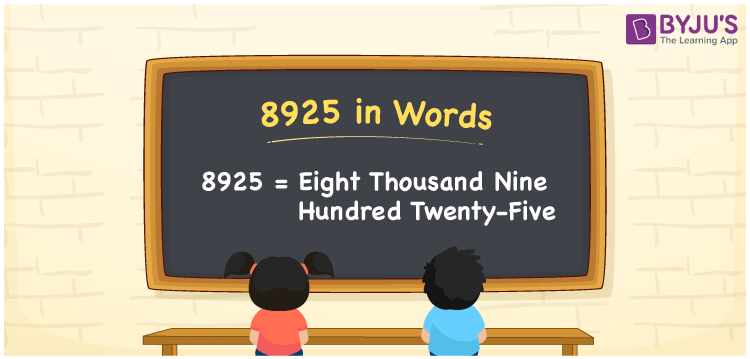# 8925 in Words

We can write 8925 in words as Eight thousand nine hundred twenty-five. If you got a compensation of Rs. 8925 in the office, you can say, “I got a compensation of Eight thousand nine hundred twenty-five rupees in the office”. It is effortless to transform the number 8925 to words using a place value chart. Also, we know that 8925 is a cardinal number since it denotes a specific quantity or amount.

 8925 in words Eight thousand nine hundred twenty-five Eight thousand nine hundred twenty-five in Numbers 8925

## 8925 in English Words

We generally write numbers in words using the English alphabet. So, we spell 8925 in English as “Eight thousand nine hundred twenty-five”.## How to Write 8925 in Words?

Let us make a place value chart with four columns to convert the number 8925 to word form, as given below.

 Thousands Hundreds Tens Ones 8 9 2 5

Here, ones = 5, tens = 2, hundreds = 9, and thousands = 8.

By expanding these numbers with respect to the place value chart, we get;

8 × Thousand + 9 × Hundred + 2 × Ten + 5 × One

= 8 × 1000 + 9 × 100 + 2 × 10 + 5 × 1

= 8000 + 900 + 20 + 5

= Eight thousand + Nine hundred + Twenty + Five

= Eight thousand nine hundred twenty-five

Therefore, 8925 in words is written as Eight thousand nine hundred twenty-five.

As we know, 8925 is a natural number that precedes 8925 and succeeds 8924.

8925 in words – Eight thousand nine hundred twenty-five

Is 8925 an even number? – No

Is 8925 an odd number? – Yes

Is 8925 a perfect square number? – No

Is 8925 a perfect cube number? – No

Is 8925 a prime number? – No

Is 8925 a composite number? – Yes

## Frequently Asked Questions on 8925 in Words

Q1

### How to write 8925 in words?

We can write the number 8925 in words as Eight thousand nine hundred twenty-five.
Q2

### What is the number name of 8925?

The number name of 8925 is “Eight thousand nine hundred twenty-five”.
Q3

### How do you write an amount of Rs. 8925 in words?

An amount of Rs. 8925 can be written in words as “Eight thousand nine hundred twenty-five rupees”.